# Metric geometry : Problem of Apollonius : rcc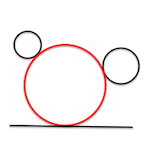Any of the problems of tangents that are included under the denomination of “Apollonius problems” can be reduced to one of the studied variants of the most basic of all: the fundamental problem of tangents (PFT).

In all these problems we will consider fundamental objective to reduce the problem to propose to one of these critical cases, by changing the constraints that define other concepts based on the orthogonality.

In this case we will study what we call “Case Apollonius RCC“, namely, For the problem of tangency at which the data are given by condition of tangency to a line (r) and two circles (cc).

We can thus stated the problem as follows:

Determining circumferences are tangent to a line and two circles

The problem has up to four possible solutions, aspect that should be analyzed in detail for one that meets design conditions that apply in each case.

Suppose that the data of the problem are determined by the C1 and C2 centers O1 and O2 circumferences, and the line r, as shown in the previous figure.

By studying the Investment in the plane saw that could transform straight in circles taking investment centers in points on the circumference.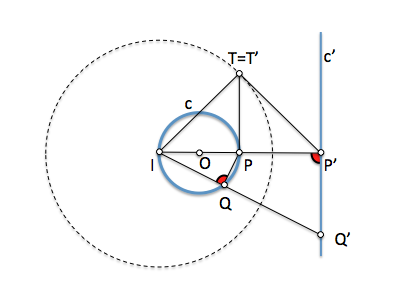The radius of the circle of self inverting (IT) we get from the power of investment IP*IP’ = IQ*IQ’ = IT*IT’ applying the constructions, for example, we have seen in the Theorem catheter.

Suppose the circumference “c” is one of the solutions sought, tangent to the circumference c1. It invertimos c1 c and one of the center points c1 (the I1), will continue to be the inverse tangent circles since the transformation is consistent. Circumference c1 will become a straight line as I1 is about c1.

If we choose the power so that c either double, c=c’, transform the line c1 is tangent to c, and circumference c=c’ will be orthogonal to the circumference of self inverting.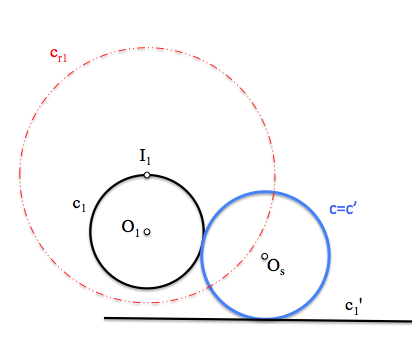This analysis allows us to obtain the orthogonality constraints to be used in our problem, considering investments positive power that exist between the circles and straight.

In our case centers I1 and I2 centers can be considered to transform the circumferences investment c1 and c2 in the straight r.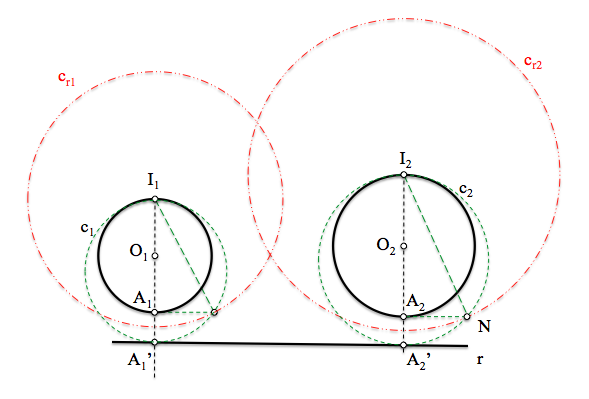In each of these transformations, circumferences are looking, solutions, be double circles and therefore they must be orthogonal to the self-inverting.

The problem can be stated from the new self-inverting circles, since they must be orthogonal to them:

Identify two orthogonal circles and tangent to a straight (the circumference)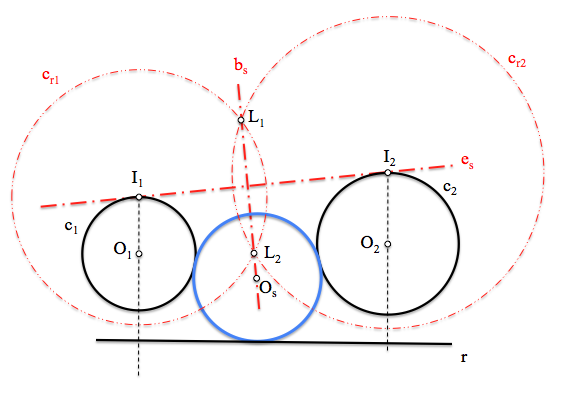This new statement is a case of the fundamental problem of tangents, since the two orthogonal circles given that belong to the conjugate beam determine. In this case, conjugate beam will be determined by the limits L1 and L2 points located on the base line.

The solution will be determined by solving the latter problem:

Determining a beam circumferences are tangent to a straight (circumference).

Metric geometry